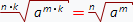Power formulas:

1. Power definition:2. Number in zero power:

a0 = 1 при условии, что a ≠ 0

a1 = a

4. Negative power transformation: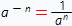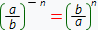5. Multiplication in power: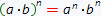if the conditions that n - is integer or a and b are real numbers and even one of them is greater than zero are hold. By using the mathematical notation this statement can be written out like this:an · am = an + m

7. Power in power: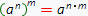8. Division in power: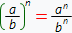9. Division with the same bases: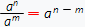10. Relationship between powers and logarithms: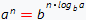1. Relationship between powers and radicals (roots):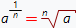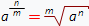2. Negative power: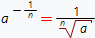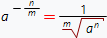3. Root from root: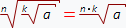4. Root from multiplication: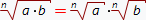5. Mutual reducement of the root and power exponents: